# 含压力基Navier-Stokes方程最优动力系统建模和分析*

(1. 大连理工大学 航空航天学院， 辽宁 大连 116024;2. 北京应用物理与计算数学研究所， 北京 100088)

(我刊编委吴锤结来稿)

## 1 含压力基的不可压缩Navier-Stokes方程的最优动力系统建模

### 1.1 含压力基的最优动力系统建模理论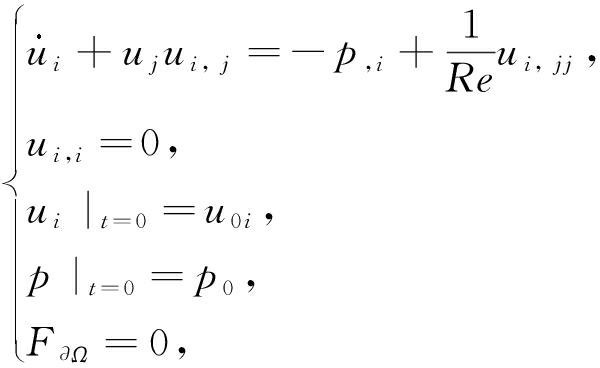(1)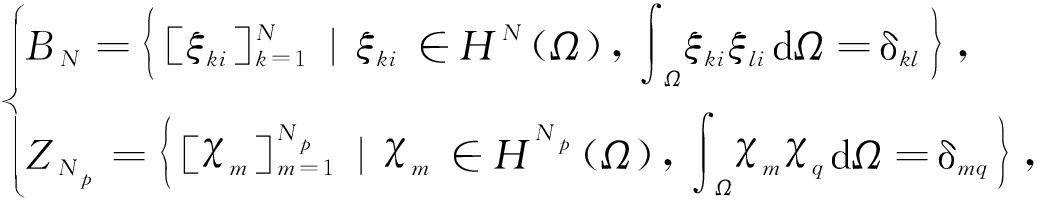(2)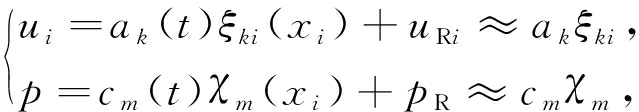(3)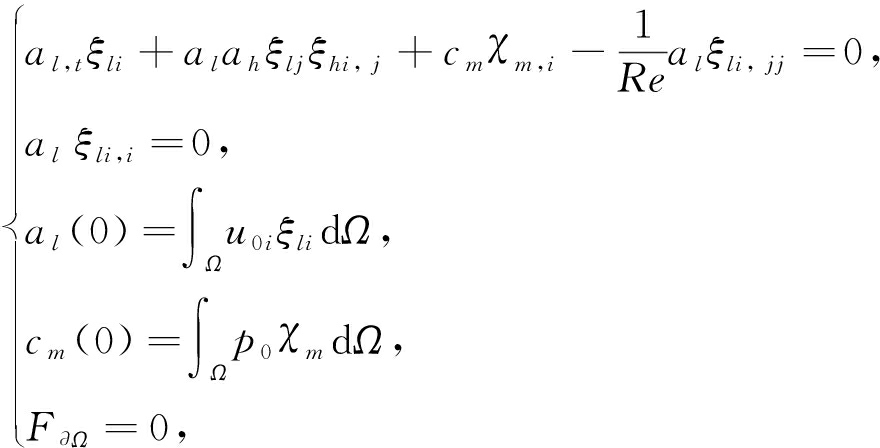(4)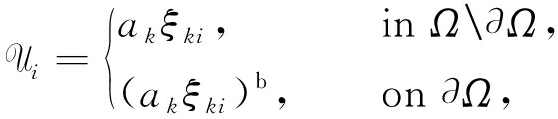(5)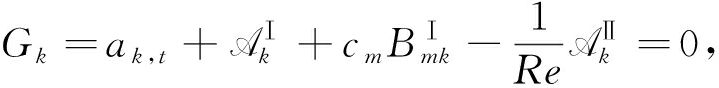(6)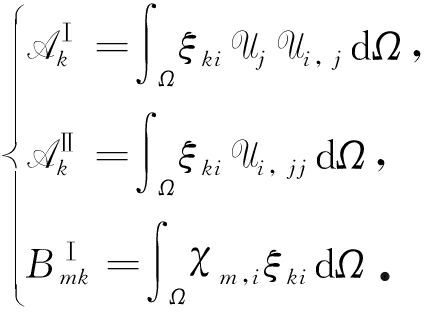(7)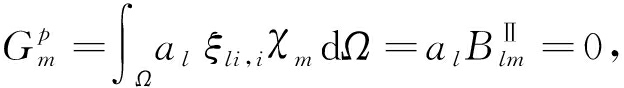(8)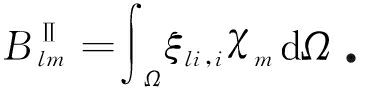(9)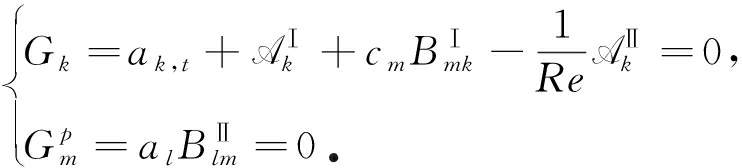(10)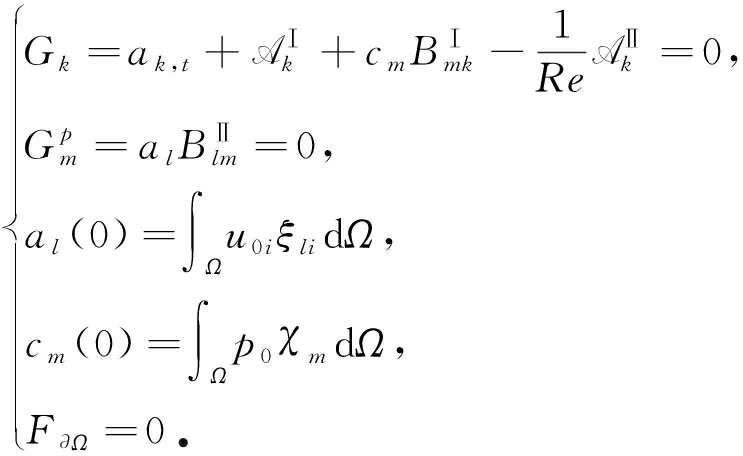(11)

### 1.2 含压力基最优动力系统模型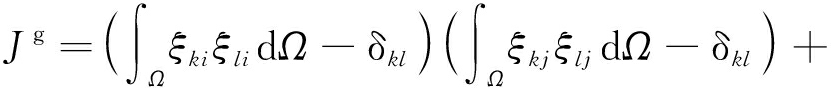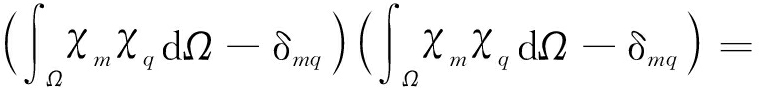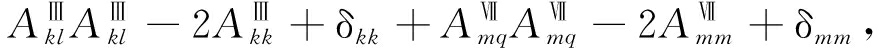(12)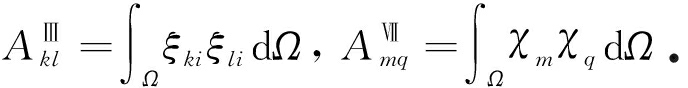(13)

1) 关于ak,t的含压力基的动力系统方程(14)

2) 广义目标泛函梯度方程

Ω内，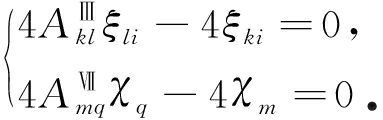(15)

### 1.3 压力基-速度基耦合动力系统方程组求解方法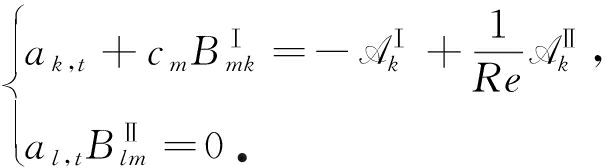(16)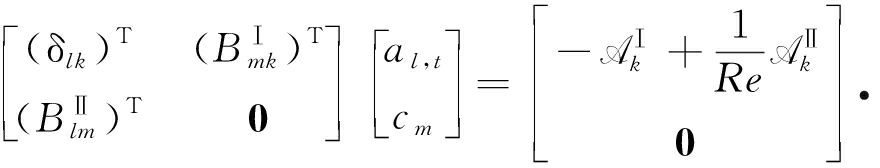(17)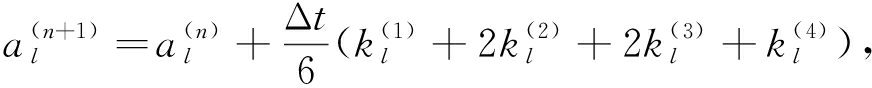(18)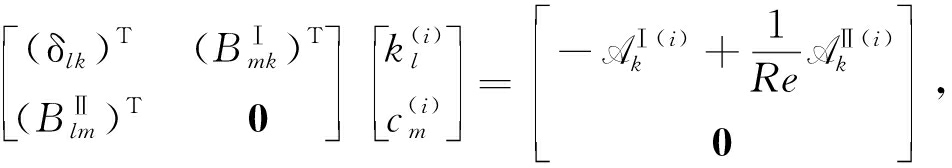(19)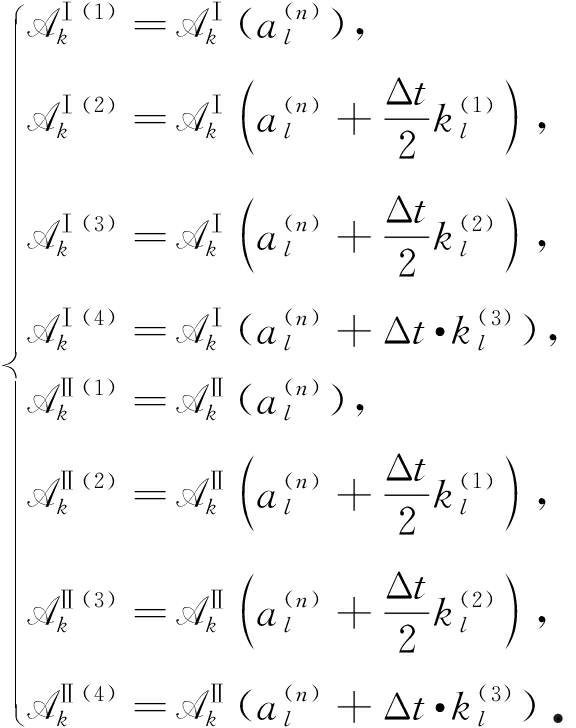(20)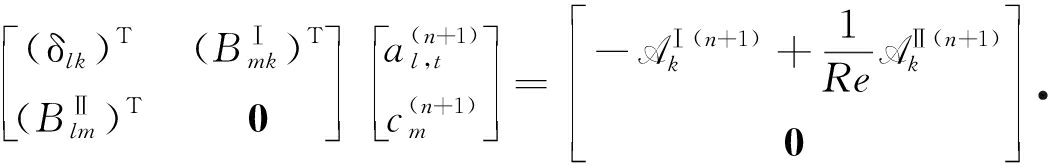(21)

### 1.4 边界条件

1)左边界 速度为入流边界和无滑移边界的混合，压力零梯度；

2)右边界 速度为连续出流边界，压力给定；

3)前后上下边界 速度为滑移边界，压力零梯度

## 2 含压力基的最优动力系统的POD分析

### 2.1 并排三方柱绕流算例和POD分析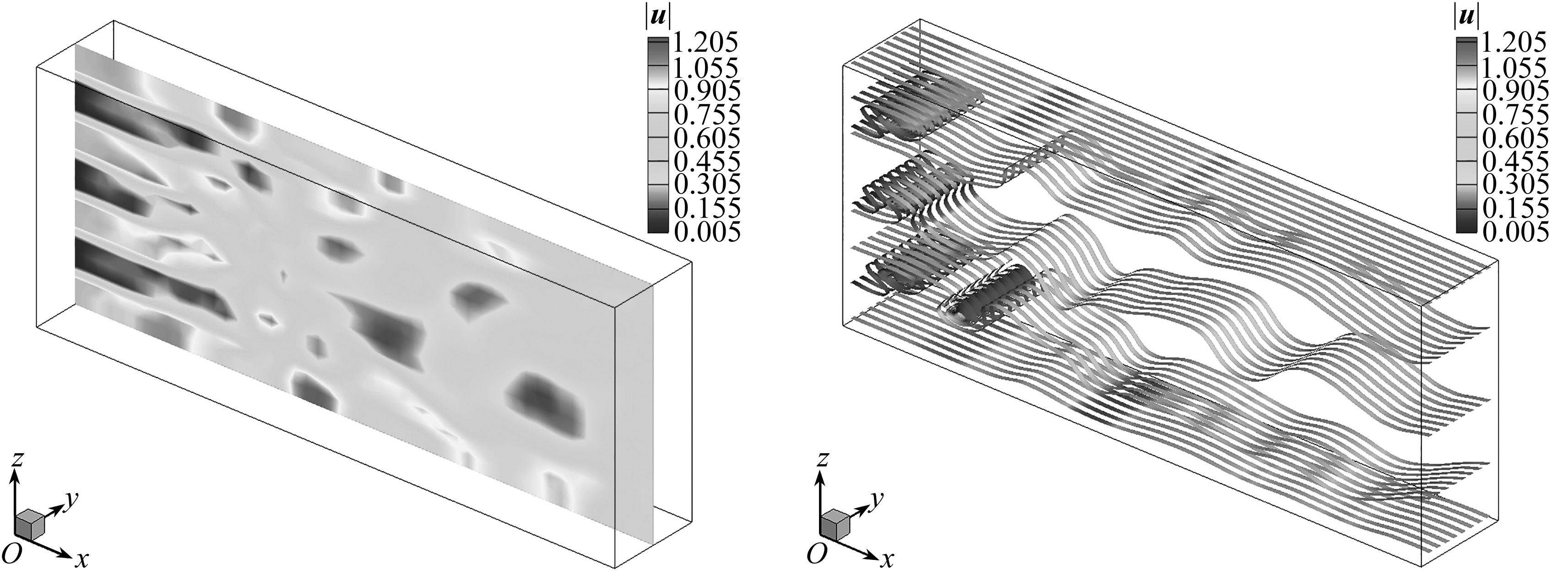(a) 速度模值云图 (b) 带状流线
(a) The contour of velocity magnitudes(b) Ribboned streamlines

Fig. 1 The coarse velocity field at t=750.0

Table 1 The energy distribution of velocity POD bases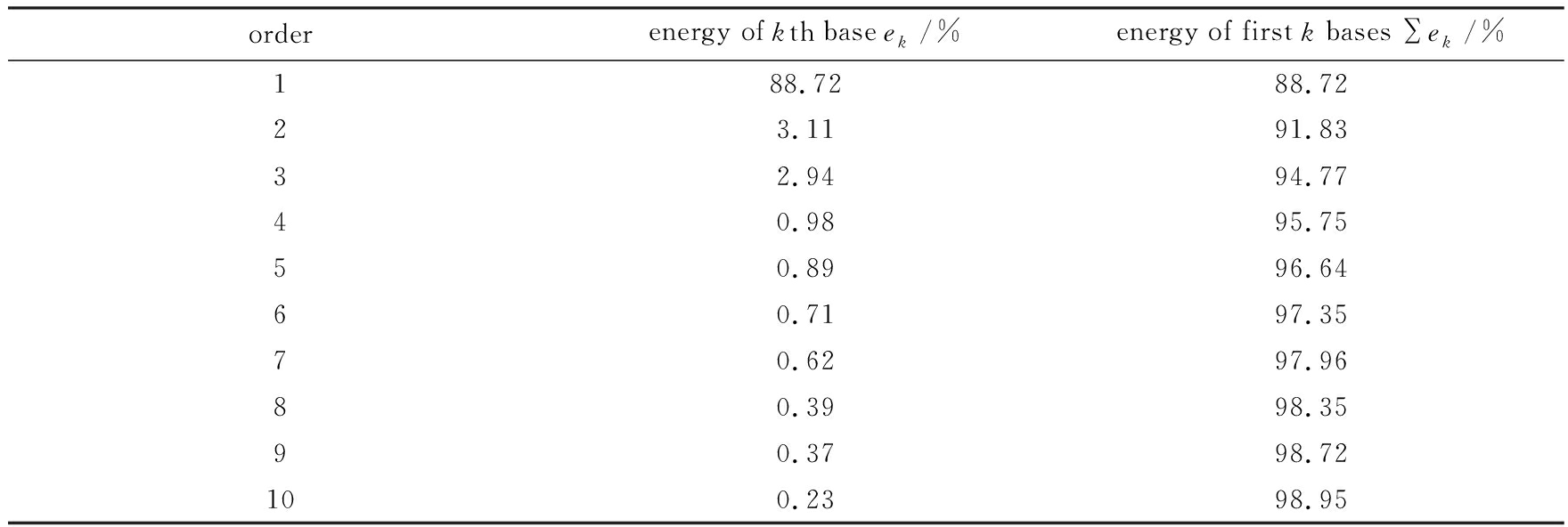orderenergy of kth base ek/%energy of first k bases ek/%188.7288.7223.1191.8332.9494.7740.9895.7550.8996.6460.7197.3570.6297.9680.3998.3590.3798.72100.2398.95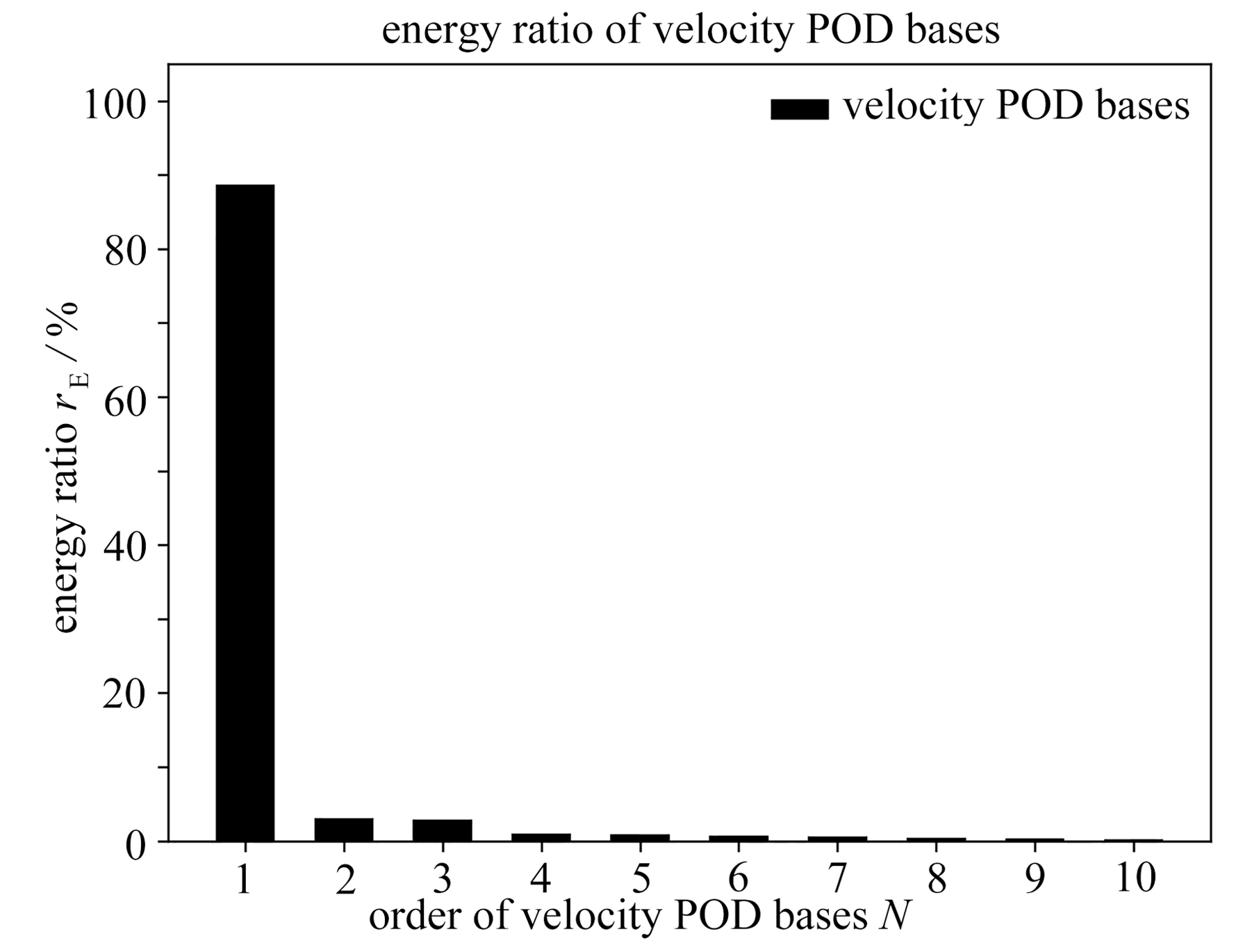Fig. 2 The energy ratios of the first 10 velocity POD bases

Table 2 The energy distribution of pressure POD bases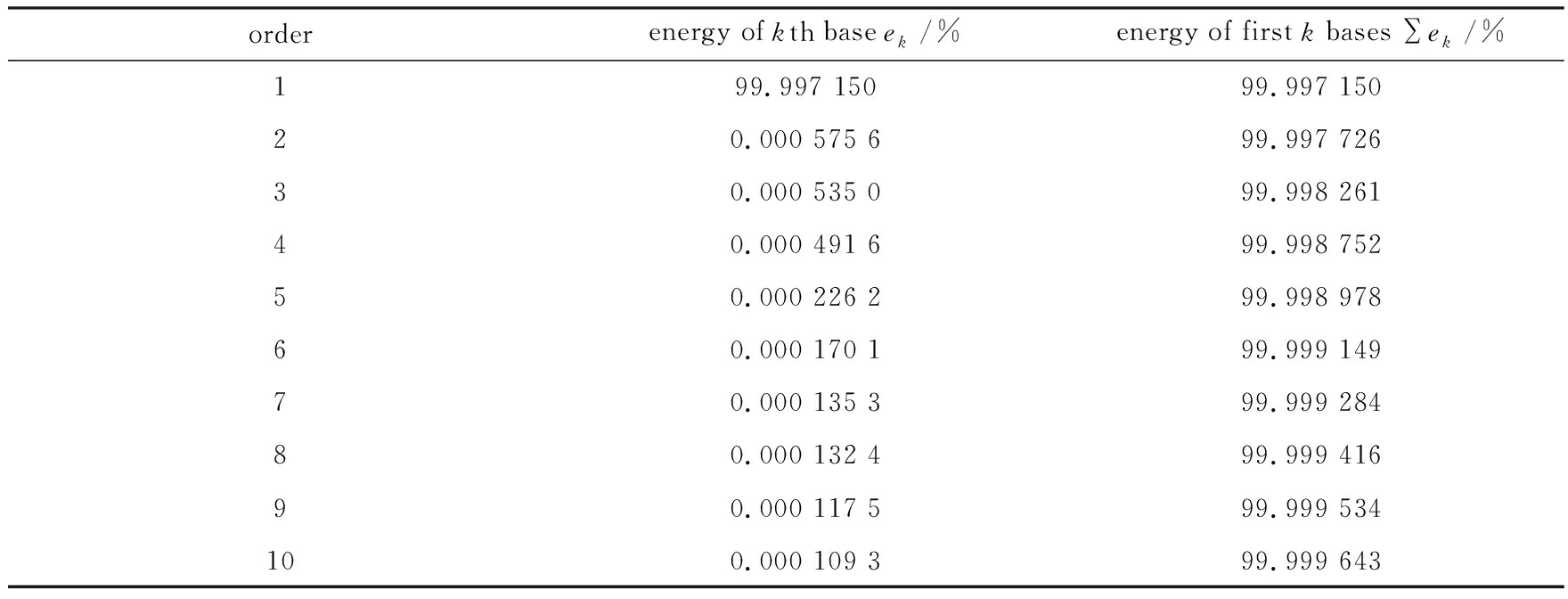orderenergy of kth base ek/%energy of first k bases ek/%199.997 15099.997 15020.000 575 699.997 72630.000 535 099.998 26140.000 491 699.998 75250.000 226 299.998 97860.000 170 199.999 14970.000 135 399.999 28480.000 132 499.999 41690.000 117 599.999 534100.000 109 399.999 643

### 2.2 近似全局最优速度基与最优压力基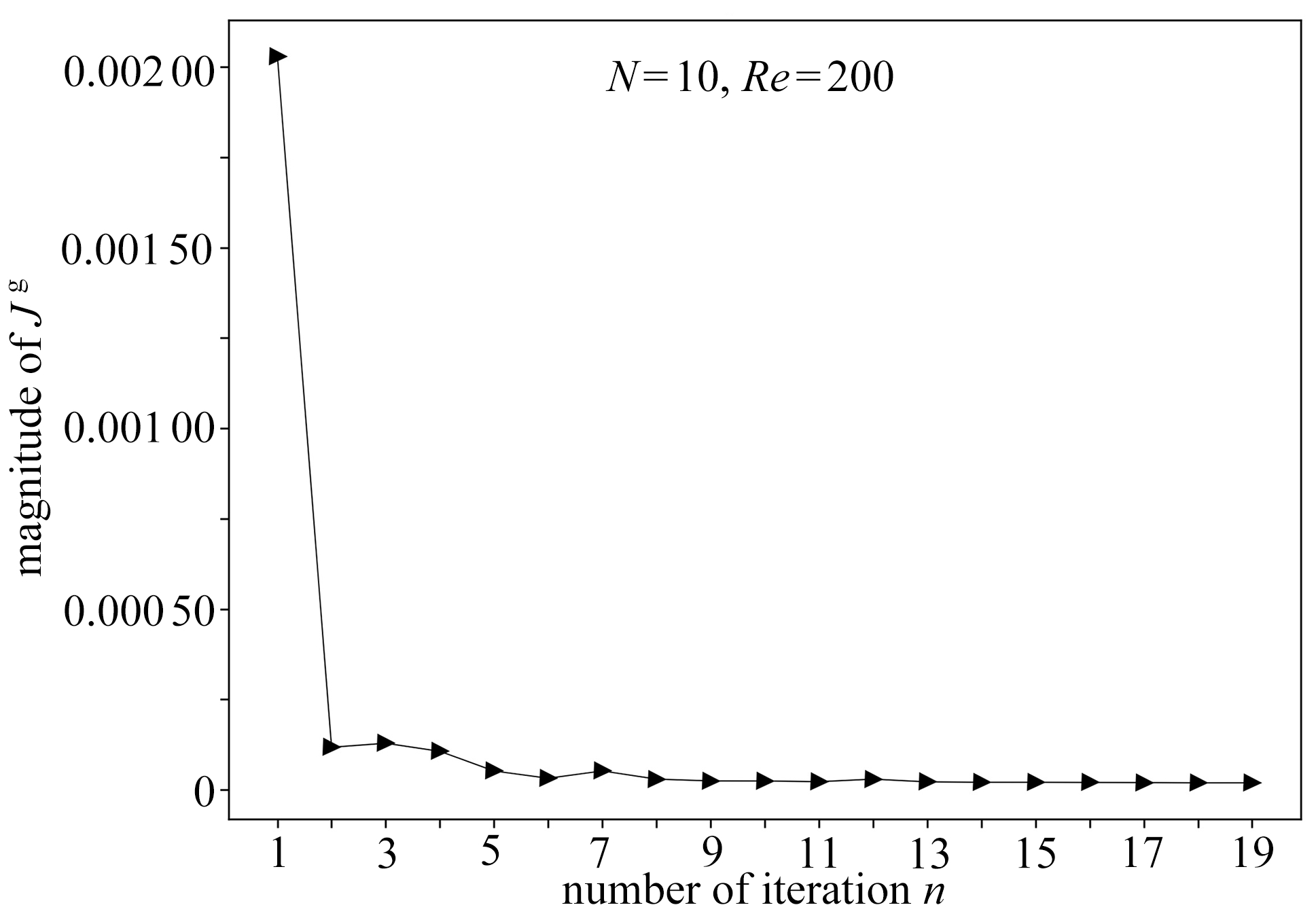Fig. 3 The convergence curve of Jg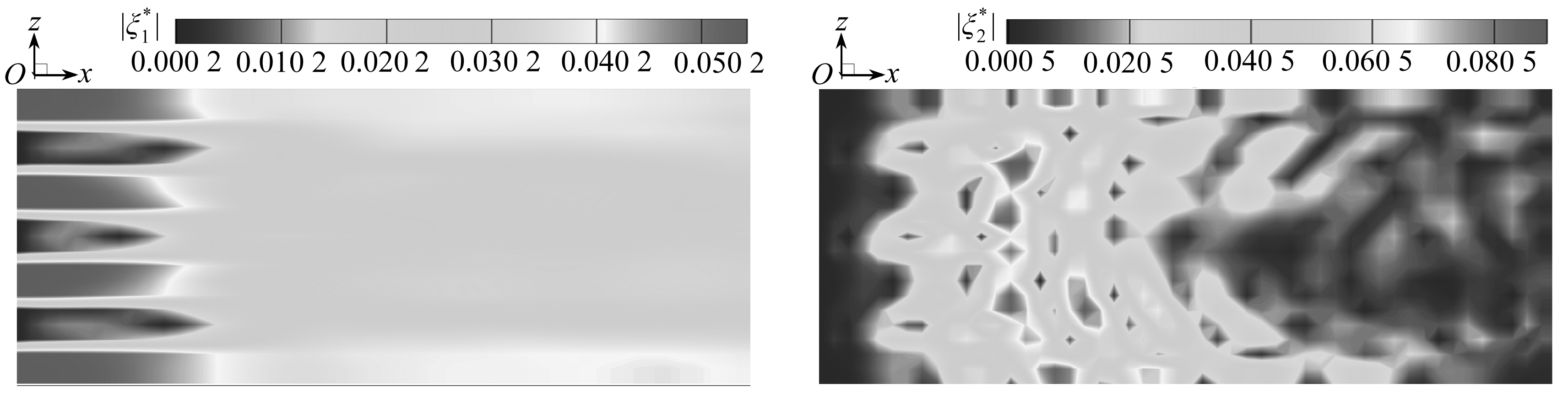(a) 第一阶 (b) 第二阶
(a) The 1st order (b) The 2nd order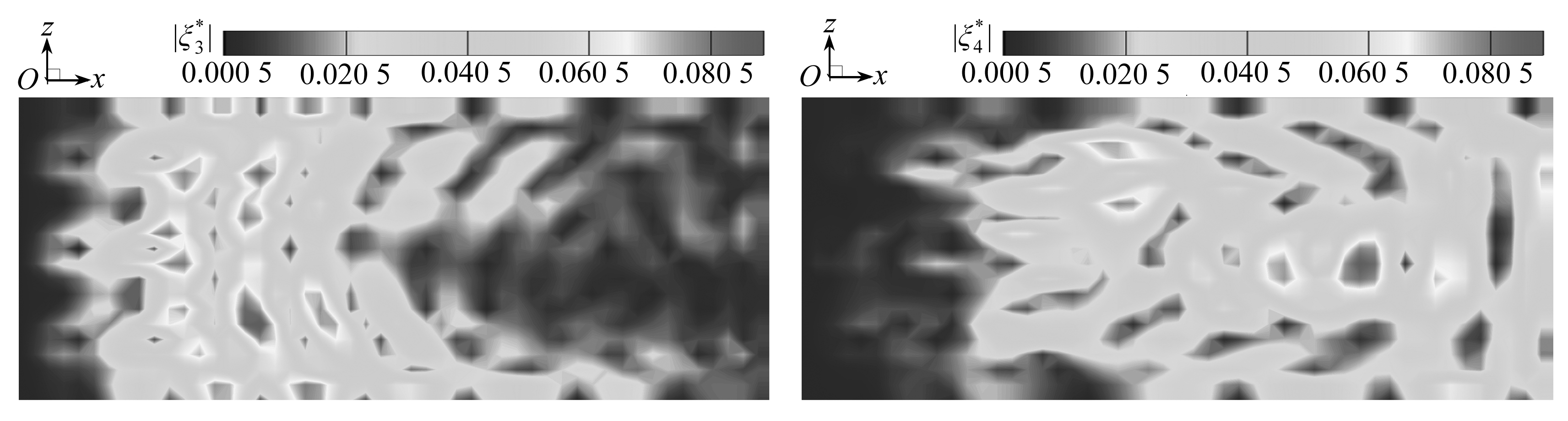(c) 第三阶 (d) 第四阶
(c) The 3rd order (d) The 4th order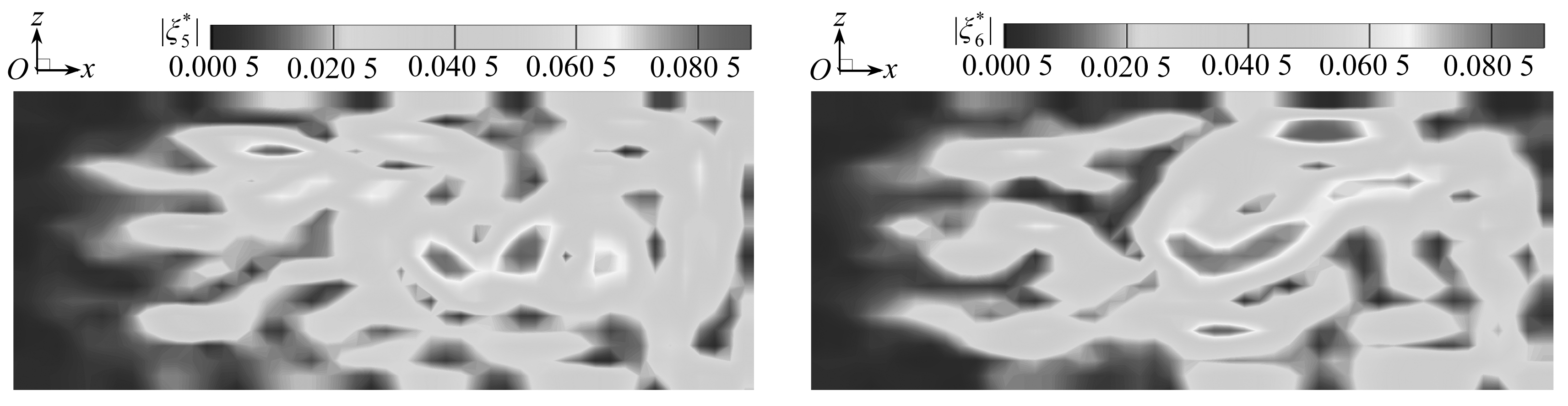(e) 第五阶 (f) 第六阶
(e) The 5th order (f) The 6th order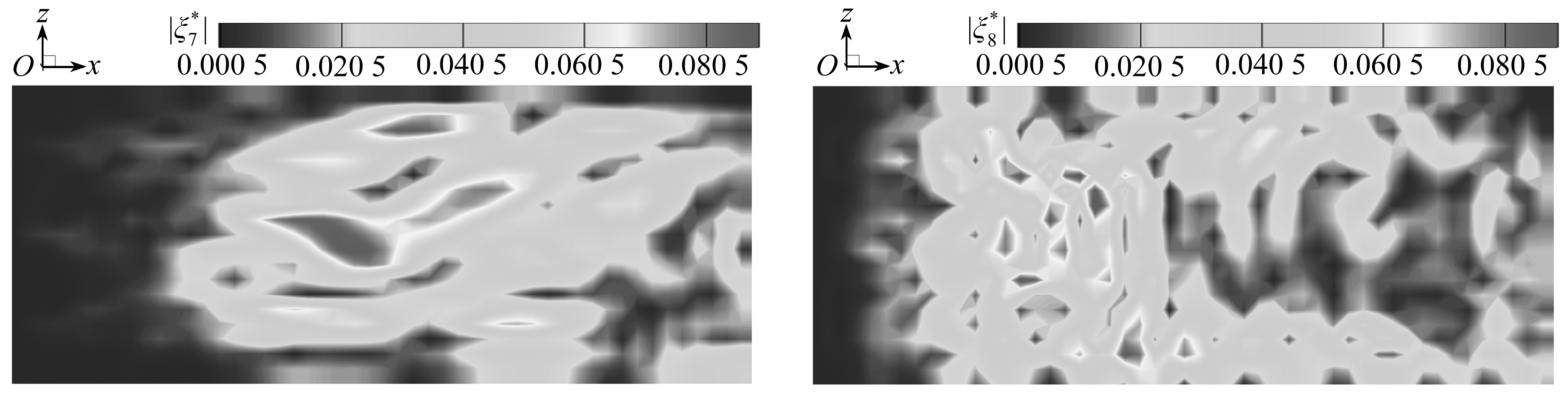(g) 第七阶 (h) 第八阶
(g) The 7th order (h) The 8th order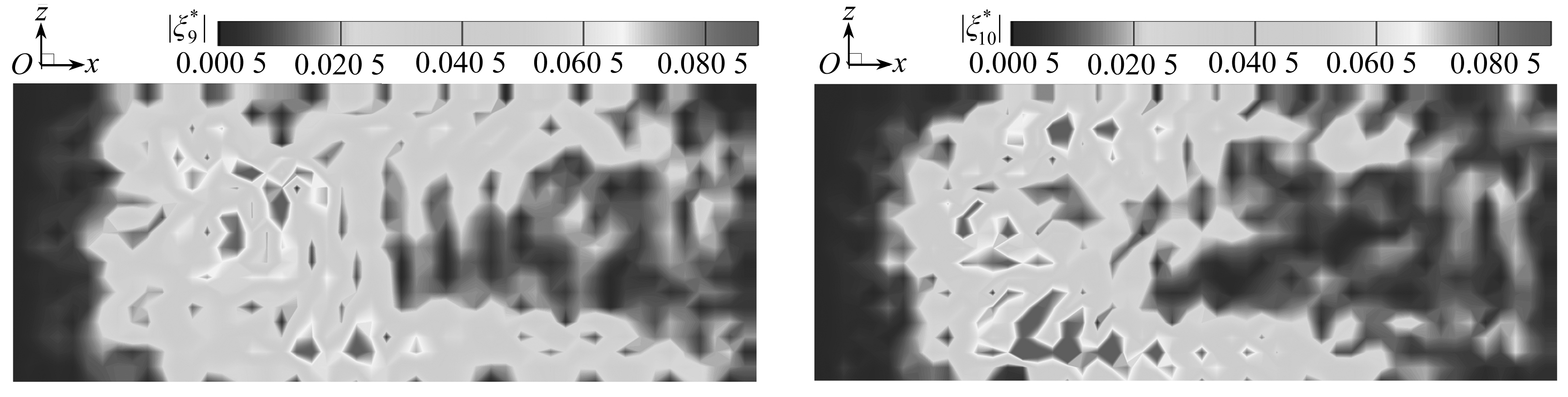(i) 第九阶 (j) 第十阶
(i) The 9th order (j) The 10th order

Fig. 4 The magnitude contours of optimal velocity bases on the streamwise middle section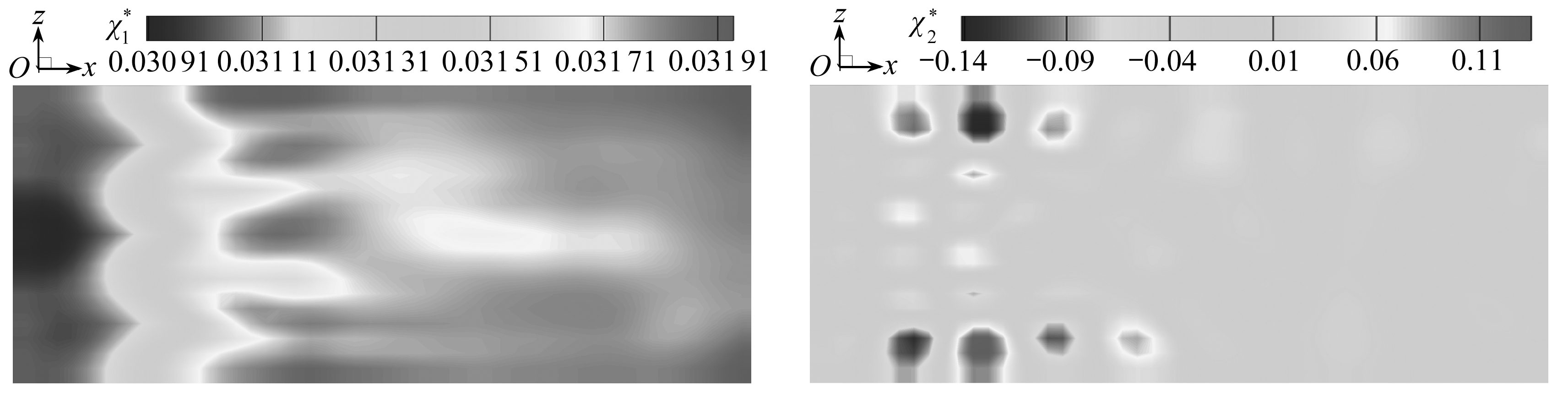(a) 第一阶 (b) 第二阶
(a) The 1st order (b) The 2nd order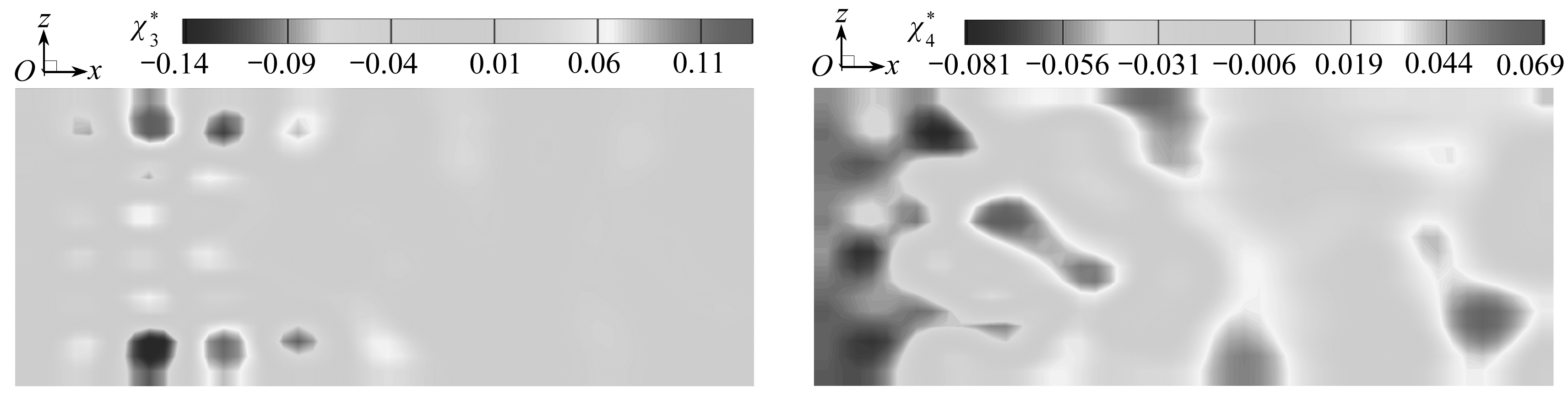(c) 第三阶 (d) 第四阶
(c) The 3rd order (d) The 4th order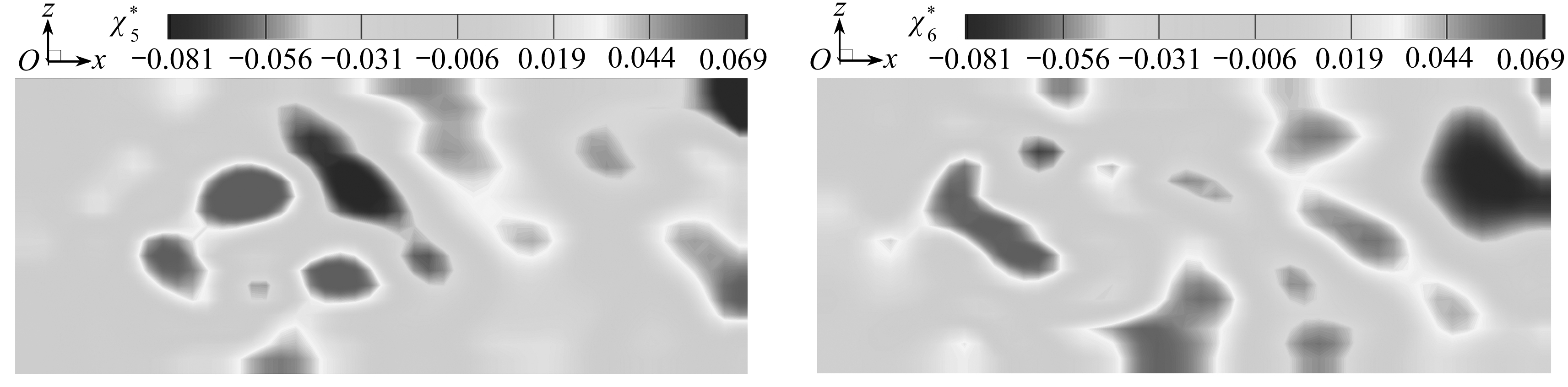(e) 第五阶 (f) 第六阶
(e) The 5th order (f) The 6th order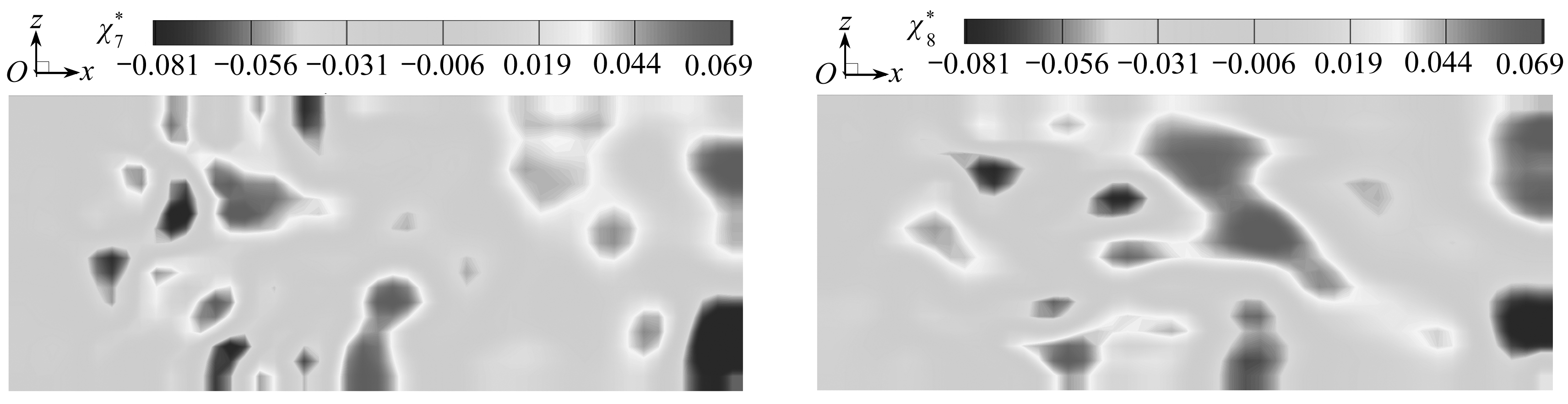(g) 第七阶 (h) 第八阶
(g) The 7th order (h) The 8th order

Fig. 5 The contours of optimal pressure bases on the streamwise middle section

### 2.3 最优基(速度基与压力基)对流场和压力场的拟合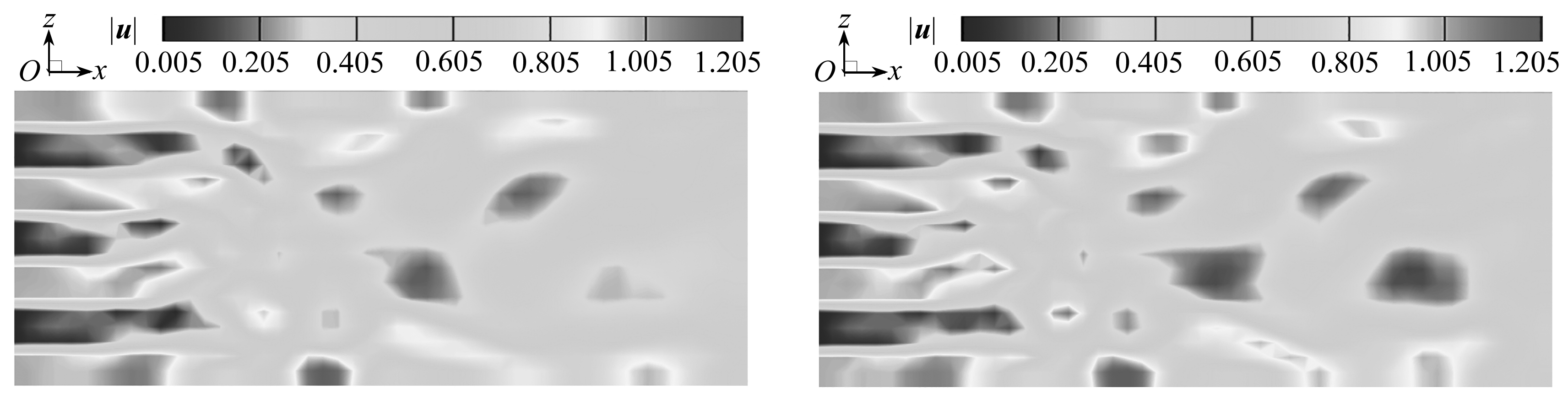(a) t=750.0最优动力系统流场 (b) t=750.0数据库流场
(a) The optimal dynamical system for t=750.0 (b) The database for t=750.0

Fig. 6 The comparison of the velocity magnitudes between the optimal dynamical system and the database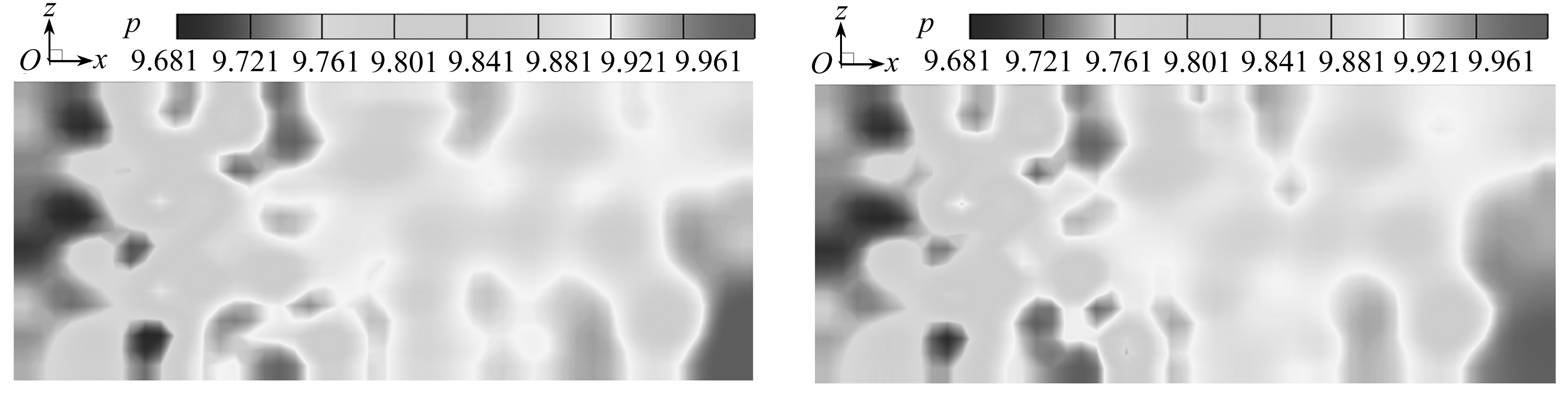(a) t=750.0最优动力系统压力场(b) t=750.0数据库压力场
(a) The optimal dynamical system for t=750.0 (b) The database for t=750.0

Fig. 7 The comparison of the pressure magnitudes between the optimal dynamical system and the database

## 3 含压力基最优动力系统的动力学特性

### 3.1 含压力基最优动力系统的相空间轨道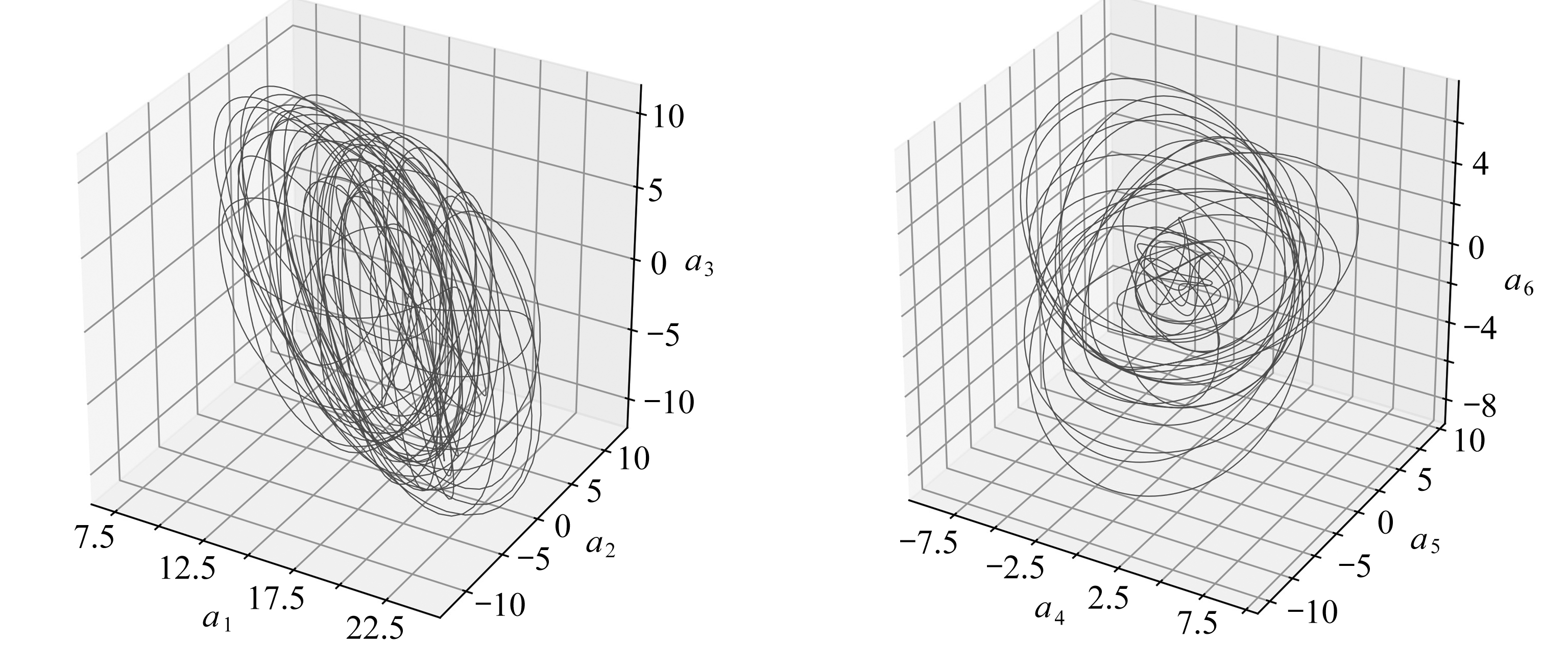(a) a1-a2-a3 (b) a4-a5-a6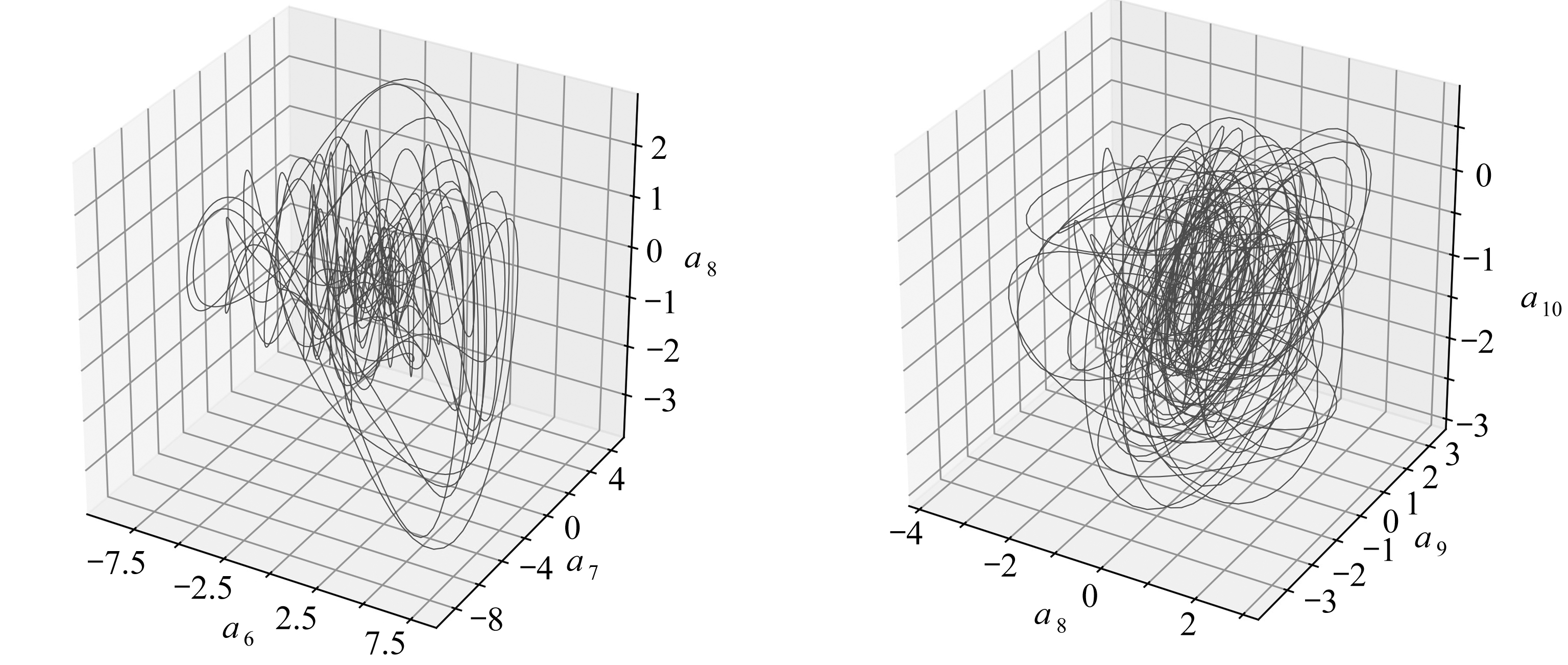(c) a6-a7-a8 (d) a8-a9-a10

Fig. 8 Three-dimensional phase portaits of the optimal dynamical systems with pressure bases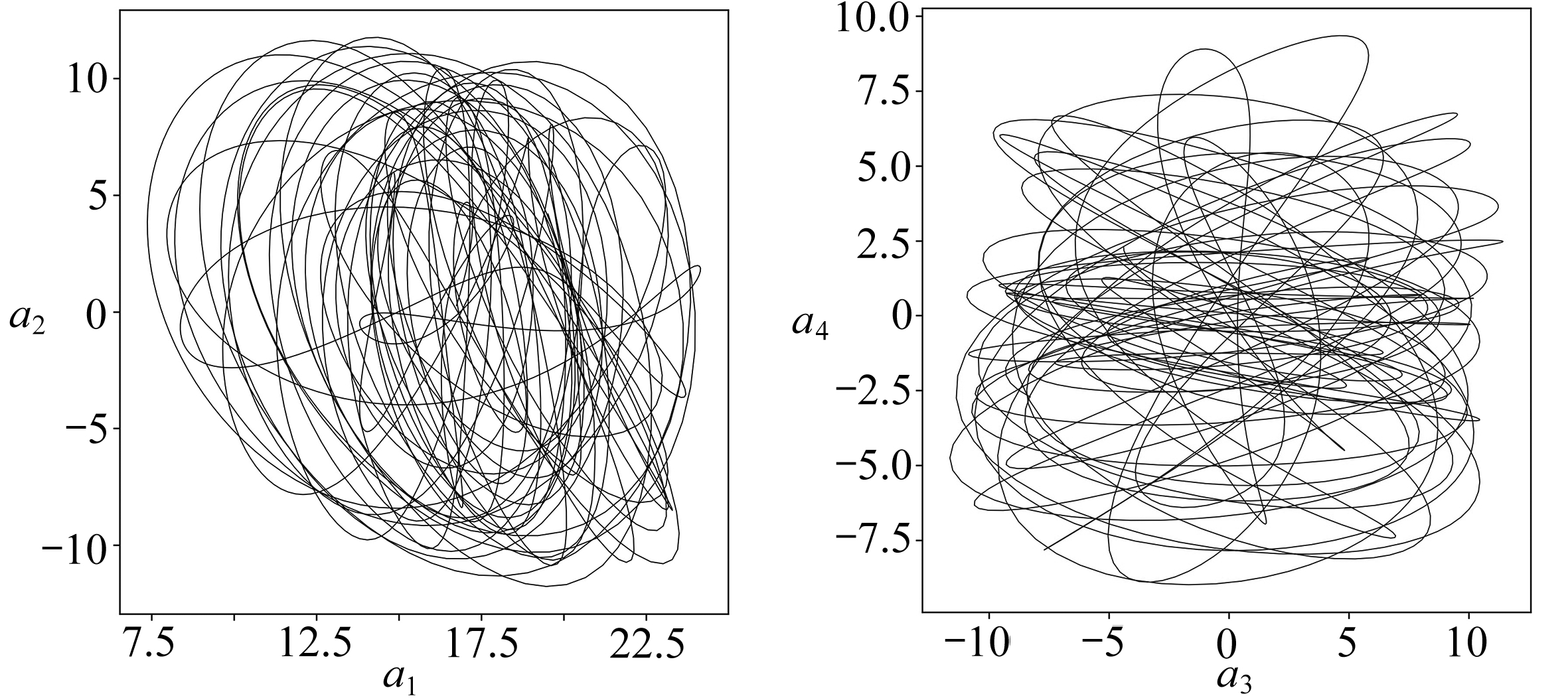(a) a1-a2(b) a3-a4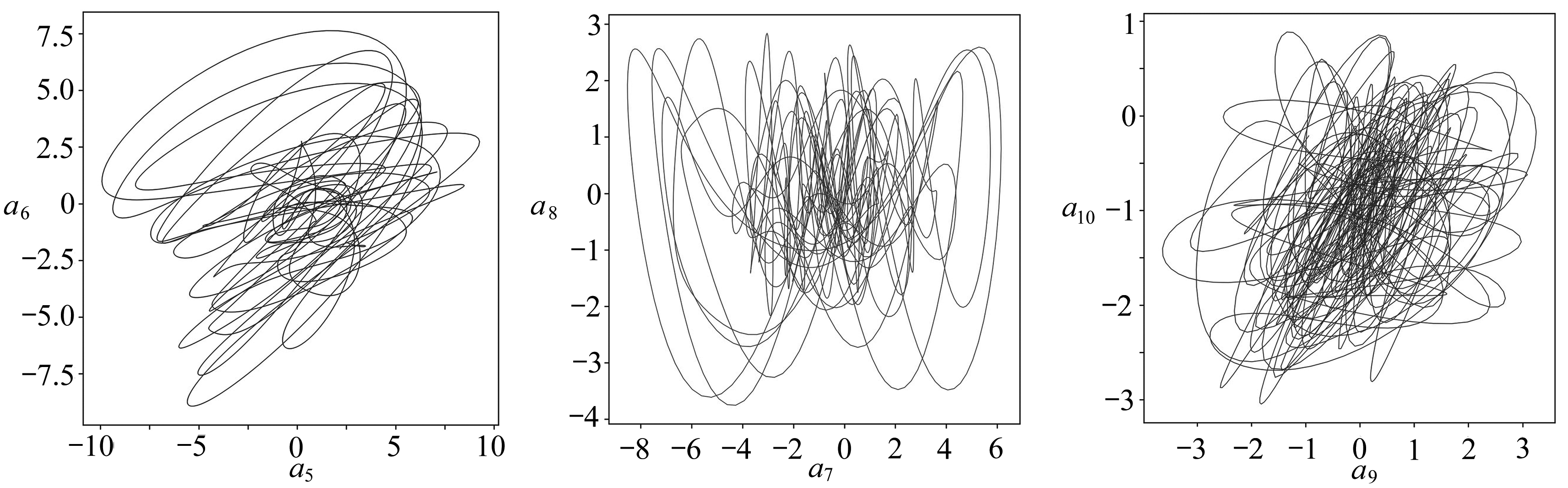(c) a5-a6(d) a7-a8(e) a9-a10

Fig. 9 Two-dimensional phase portaits of the optimal dynamical systems with pressure bases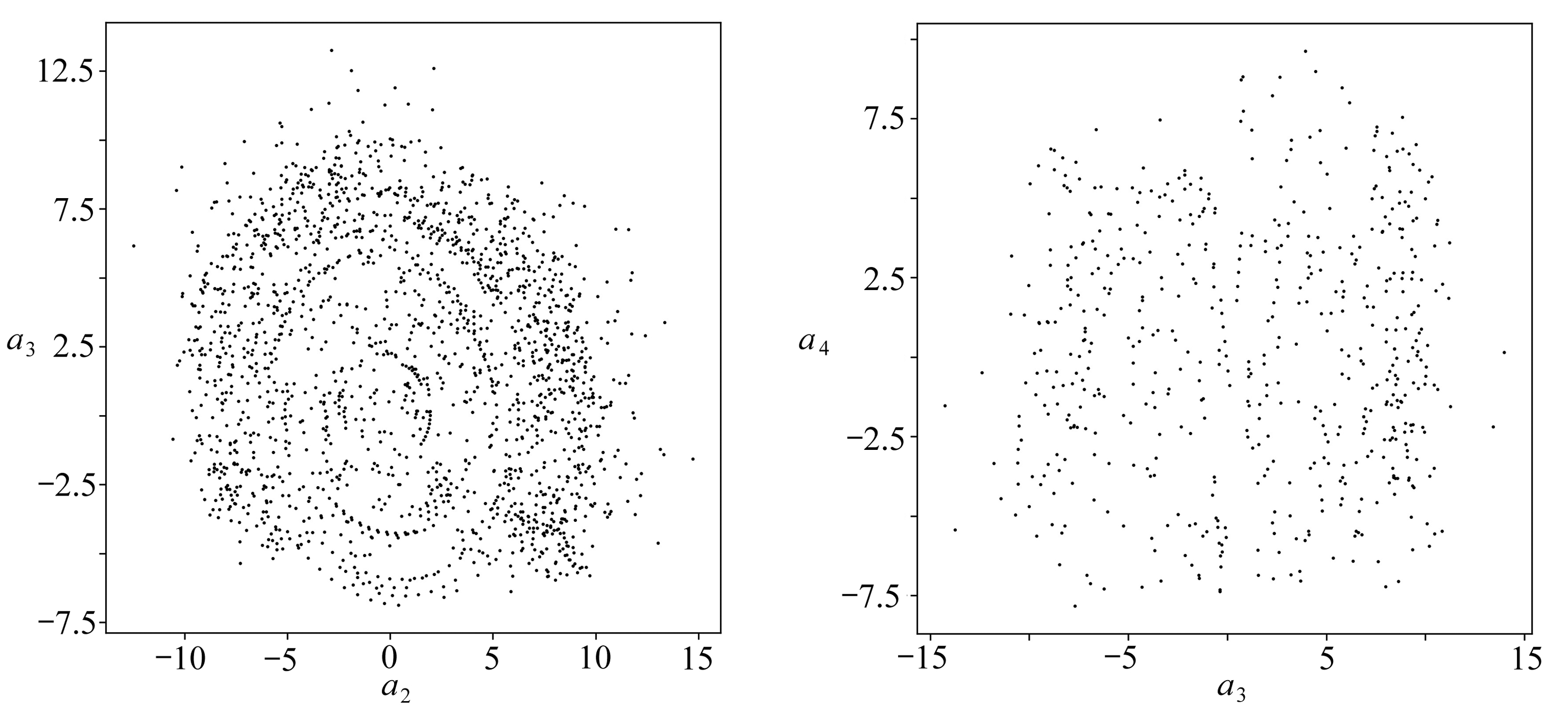(a) 相平面a1=0 (b) 相平面a2=0
(a) The phase plane of a1=0 (b) The phase plane of a2=0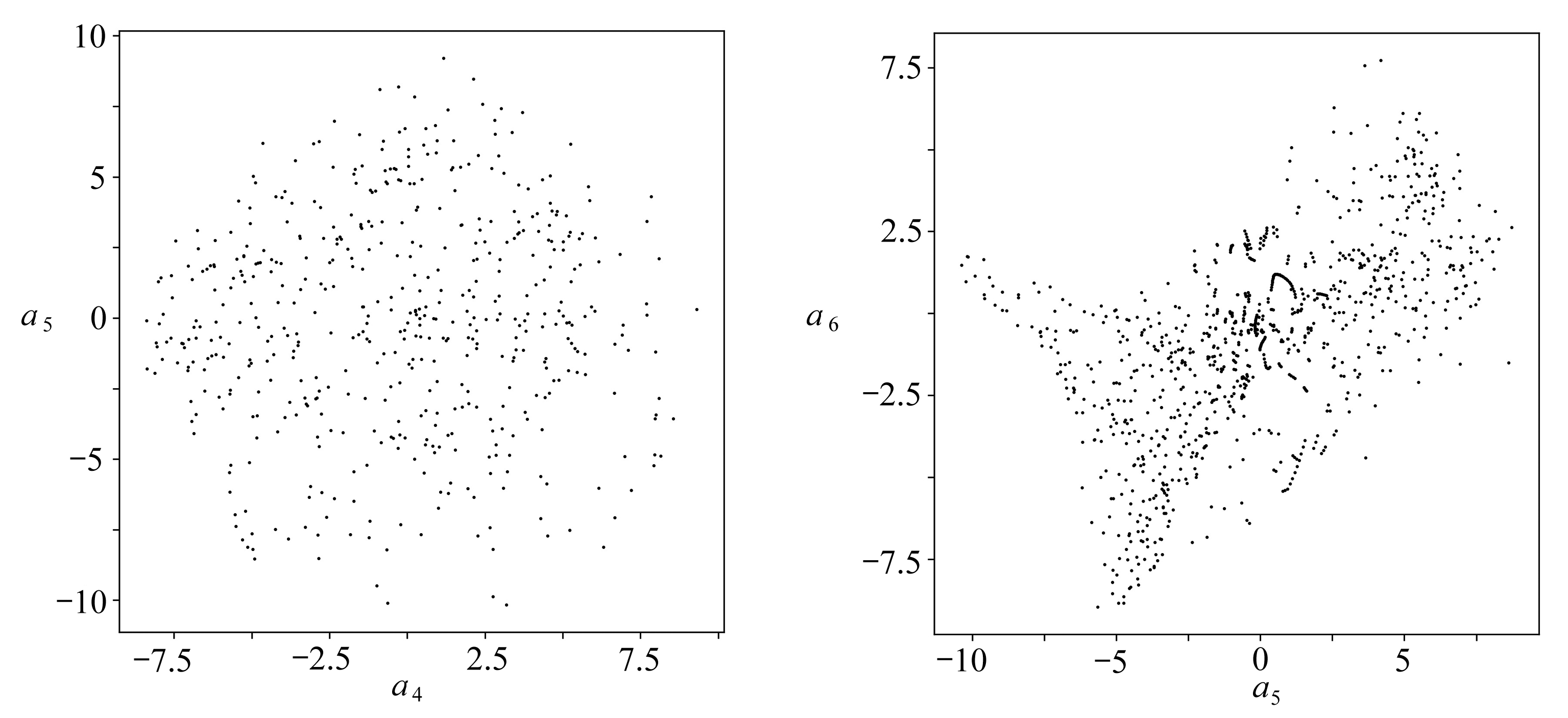(c) 相平面a3=0 (d) 相平面a4=0
(c) The phase plane of a3=0 (d) The phase plane of a4=0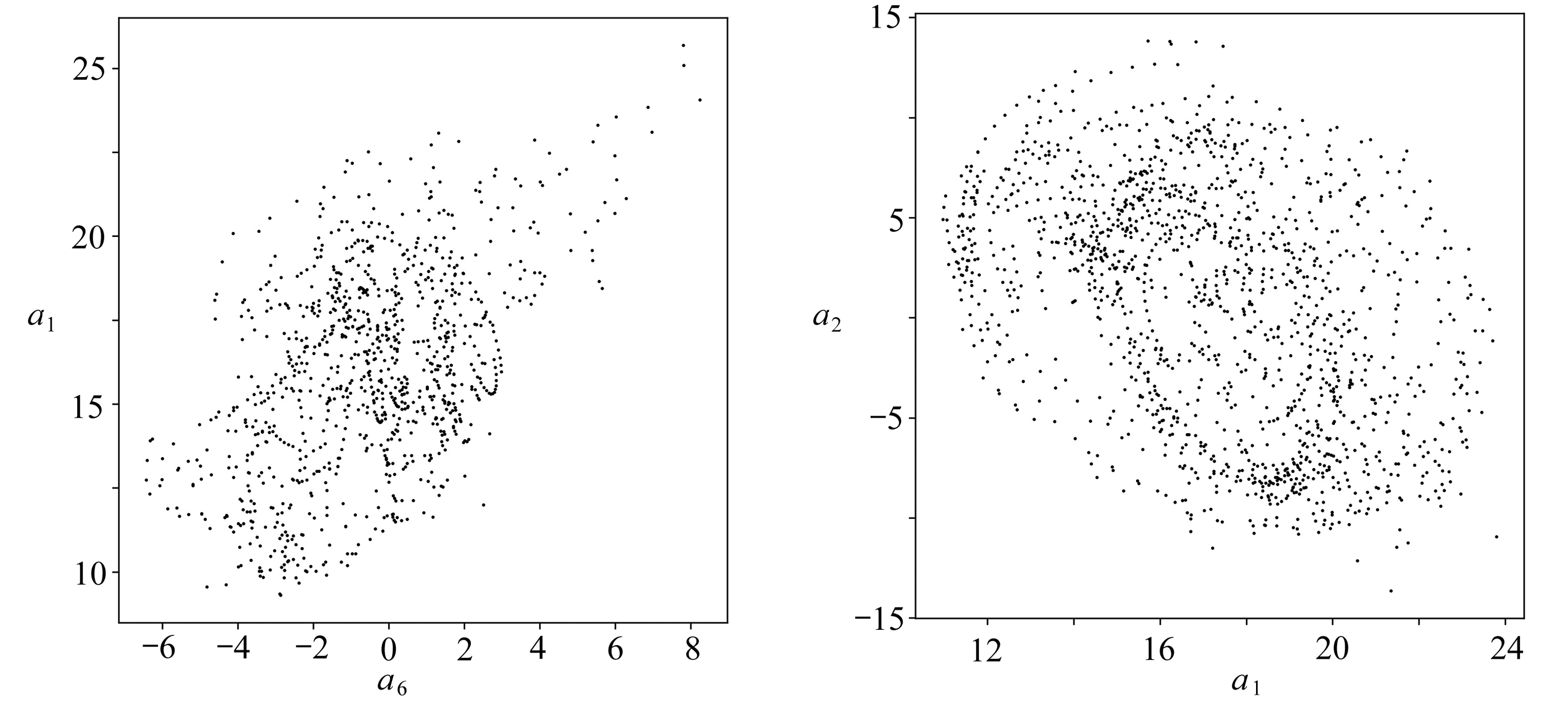(e) 相平面a5=0 (f) 相平面a6=0
(e) The phase plane of a5=0 (f) The phase plane of a6=0

Fig. 10 Six typical Poincaré sections of the optimal dynamical systems with pressure bases

### 3.2 含压力基最优动力系统的Poincaré截面

Poincaré截面可以被用于判断系统是否具有混沌特性从含压力基的最优动力系统在六个典型相平面上的Poincaré截面(图10)可见，各个Poincaré截面都是由一系列不重叠的点组成的，因此可以断定含压力基的最优动力系统在Re=200时的长期动力学特性是混沌

### 3.3 含压力基最优动力系统的分岔特性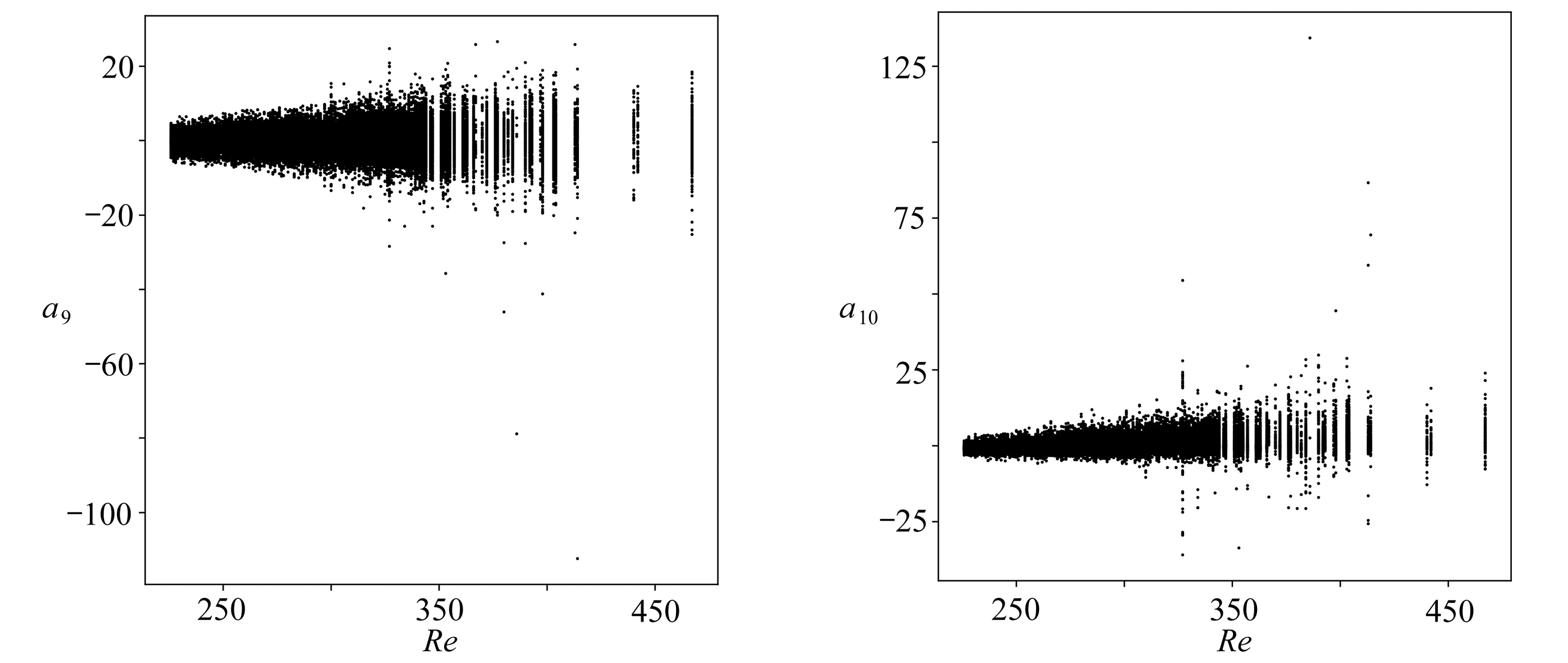(a) a9的分岔特性(b) a10的分岔特性
(a) The bifurcation characteristics of a9(b) The bifurcation characteristics of a10

Fig. 11 The bifurcation diagram of the optimal dynamical systems for Re=200～700

### 3.4 含压力基最优动力系统的功率谱分析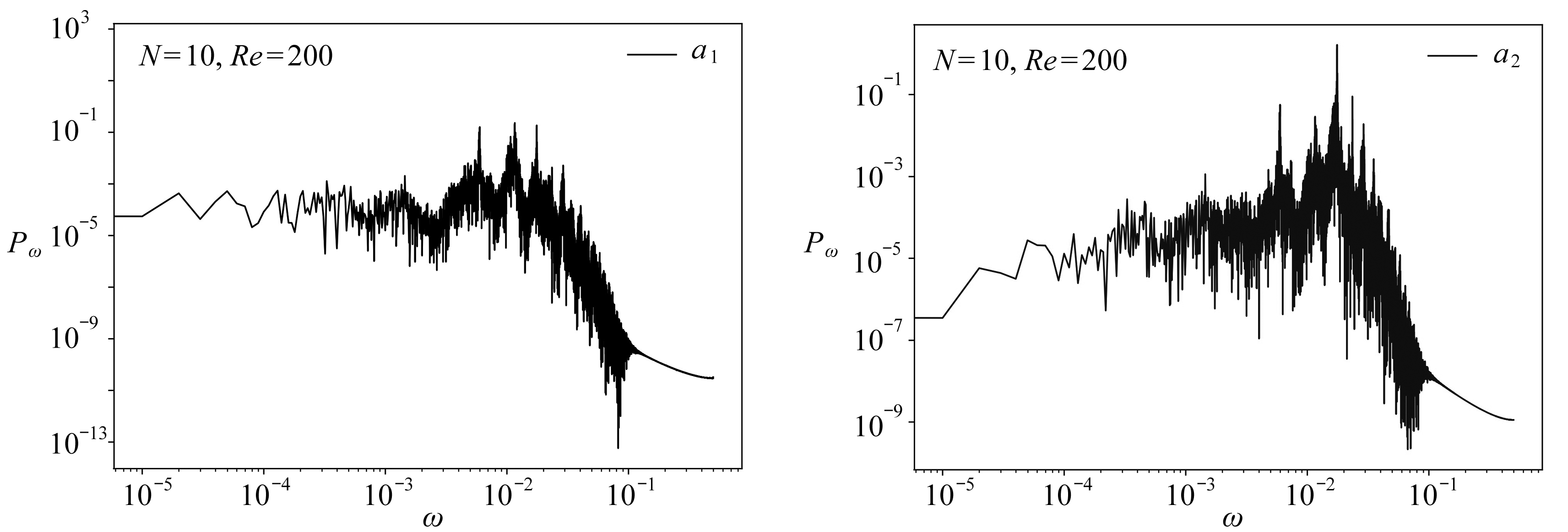(a) a1的功率谱 (b) a2的功率谱
(a) The power spectrum of a1 (b) The power spectrum of a2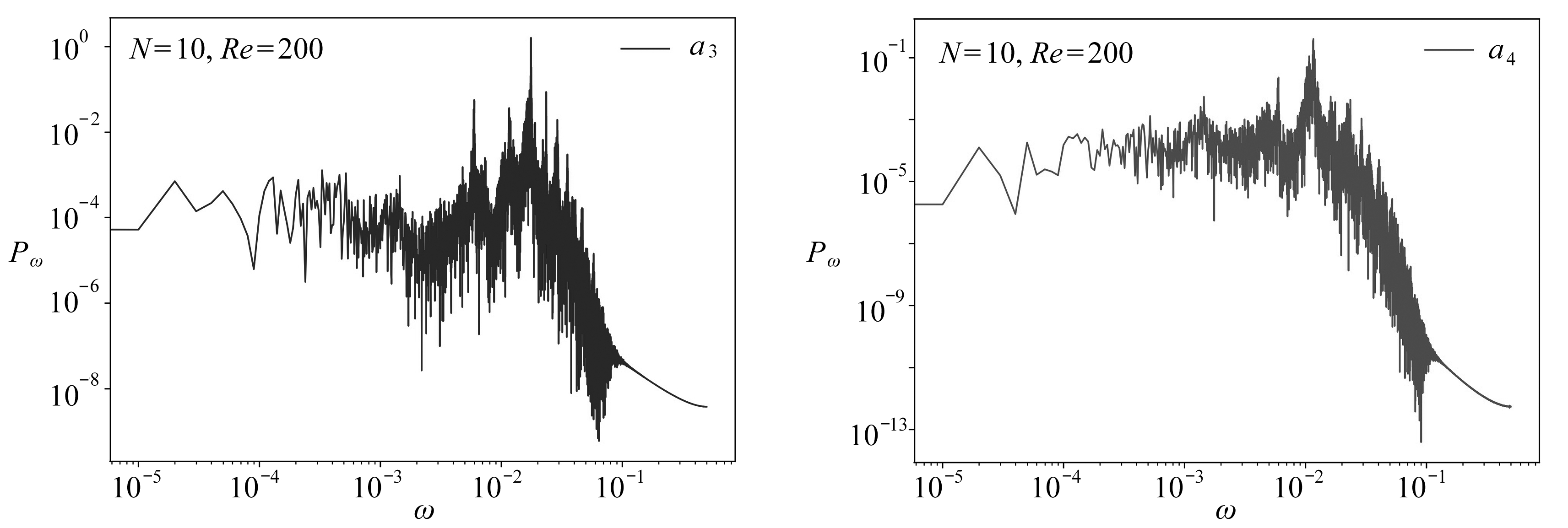(c) a3的功率谱 (d) a4的功率谱
(c) The power spectrum of a3 (d) The power spectrum of a4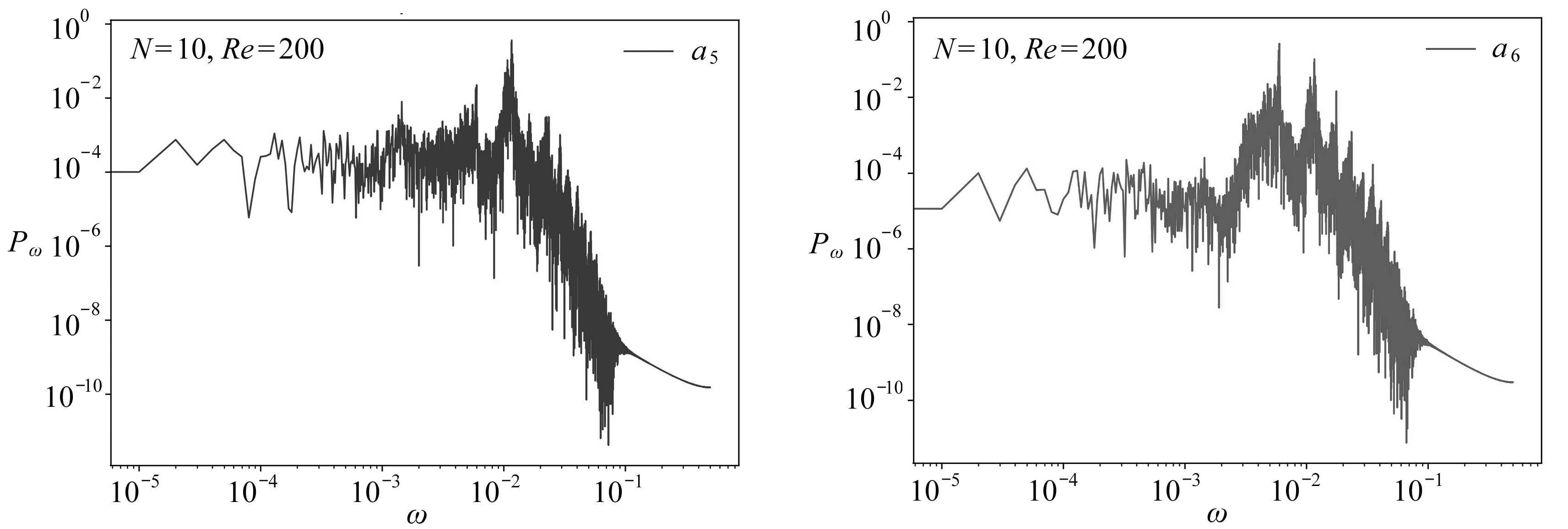(e) a5的功率谱 (f) a6的功率谱
(e) The power spectrum of a5 (f) The power spectrum of a6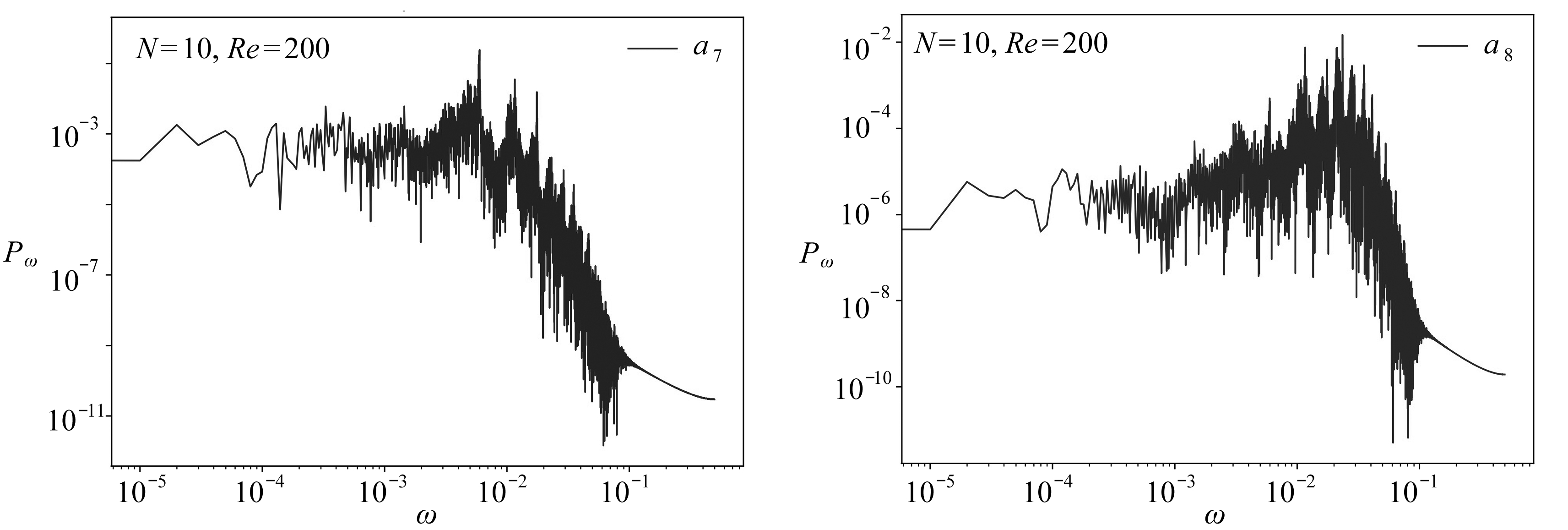(g) a7的功率谱 (h) a8的功率谱
(g) The power spectrum of a7 (h) The power spectrum of a8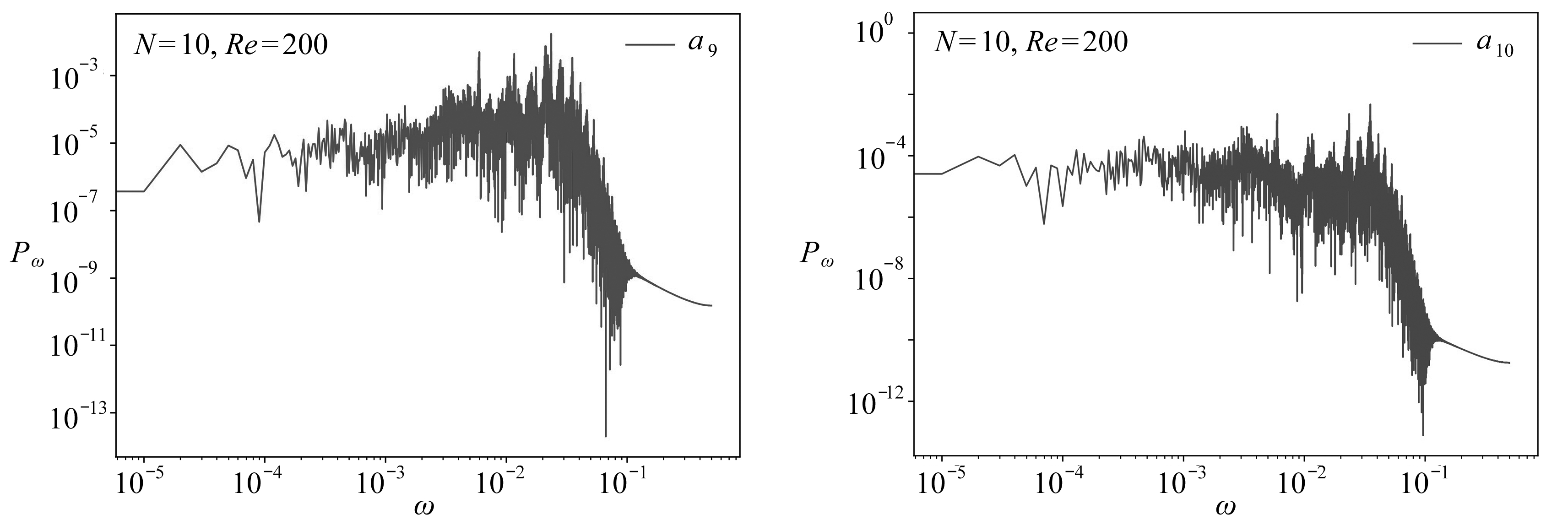(i) a9的功率谱 (j) a10的功率谱
(i) The power spectrum of a9 (j) The power spectrum of a10

Fig. 12 The power spectra of the optimal dynamical systems with pressure bases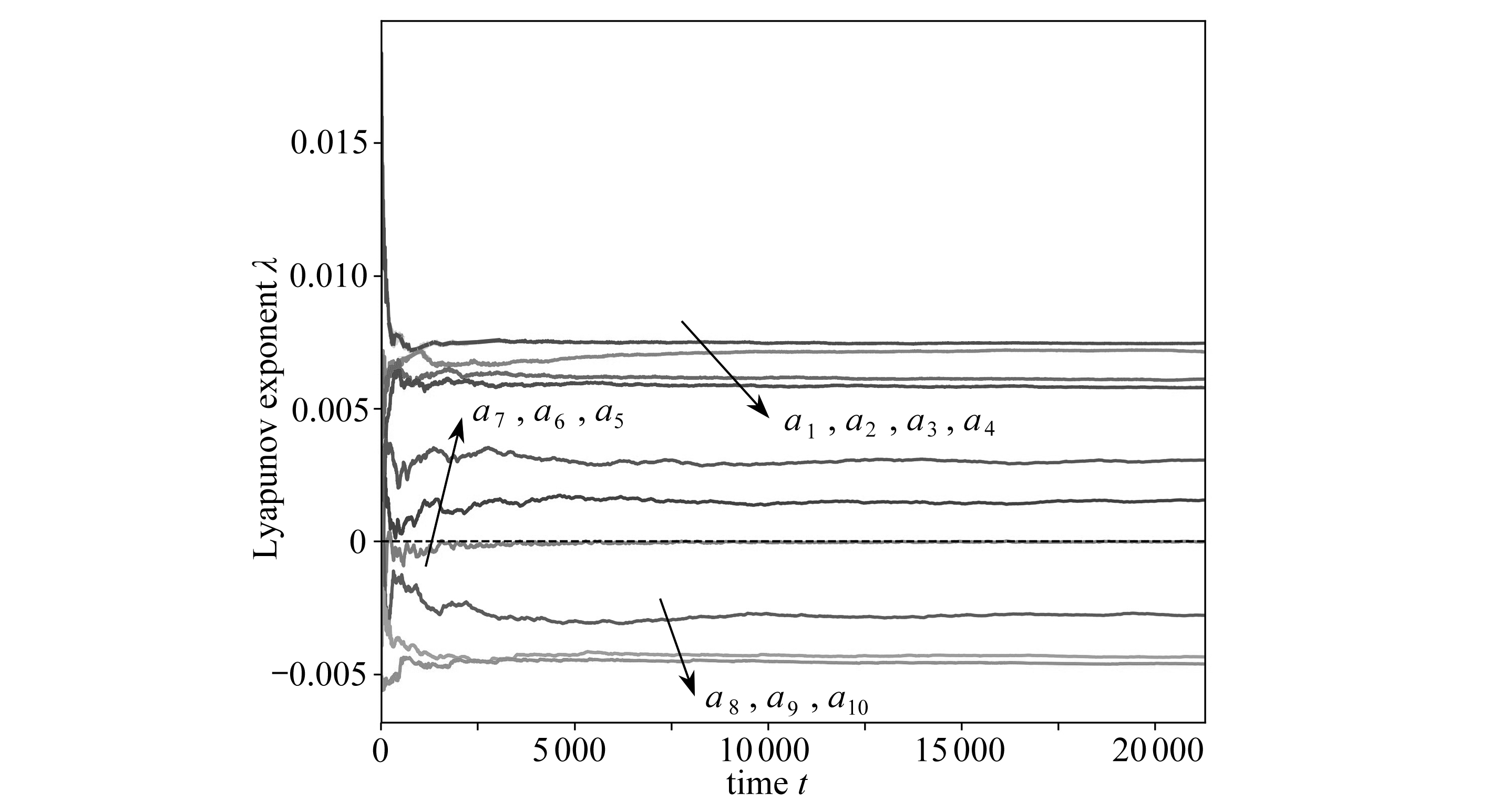Fig. 13 The evolution of Lyapunov exponent spectra of the optimal dynamical systems

### 3.5 含压力基最优动力系统的Lyapunov指数

Lyapunov指数集能够更为准确地判断动力系统的长期动力学行为

## 4 结 论

 WU C J. Optimal truncated low-dimensional dynamical systems[J]. Discrete and Continuous Dynamical Systems, 1996, 2(4): 559-583.

 WU C J, SHI H S. An optimal theory for an expansion of flow quantities to capture the flow structures[J]. Fluid Dynamics Research, 1995, 17(2): 67-85.

 吴锤结, 赵红亮. 不依赖数据库的最优动力系统建模理论及其应用[J]. 力学学报, 2001, 33(3): 289-300.(WU Chuijie, ZHAO Hongliang. A new database-free method of constructing optimal low-dimensional dynamical systems and its application[J]. Acta Mechanica Sinica, 2001, 33(3): 289-300.(in Chinese))

 WU C J, WANG L. A method of constructing a database-free optimal dynamical system and a global optimal dynamical system[J]. Science in China(Series G): Physics, Mechanics & Astronomy, 2008, 51(7): 905-915.

 PENG N F, GUAN H, WU C J. Research on the optimal dynamical systems of three-dimensional Navier-Stokes equations based on weighted residual[J]. Science China: Physics, Mechanics & Astronomy, 2016, 59(4): 644-701.

 PENG N F, GUAN H, WU C J. Optimal dynamical systems of Navier-Stokes equations based on generalized helical-wave bases and the fundamental elements of turbulence[J]. Science China: Physics, Mechanics & Astronomy, 2016, 59(11): 114713.

 王金城, 齐进, 吴锤结. Navier-Stokes方程的脉动速度方程的最优动力系统建模和动力学分析[J]. 应用数学和力学, 2020, 41(3): 235-249.(WANG Jincheng, QI Jin, WU Chuijie. Dynamics analysis and modelling of optimal dynamical systems of the fluctuation velocity equations of incompressible Navier-Stokes equations[J]. Applied Mathematics and Mechanics, 2020, 41(3): 235-249.(in Chinese))

 王金城, 齐进, 吴锤结. 不可压缩Navier-Stokes方程最优动力系统建模和分析[J]. 应用数学和力学, 2020, 41(1): 1-15.(WANG Jincheng, QI Jin, WU Chuijie. Analysis and modelling of optimal dynamical systems of incompressible Navier-Stokes equations[J]. Applied Mathematics and Mechanics, 2020, 41(1): 1-15.(in Chinese))

 黄克智, 薛明德, 陆明万. 张量分析[M]. 北京: 清华大学出版社, 2003.(HUANG Kezhi, XUE Mingde, LU Mingwan. Tensor Analysis[M]. Beijing: Tsinghua University Press, 2003.(in Chinese))

 叶庆凯, 王肇明. 优化与最优控制中的计算方法[M]. 北京: 科学出版社, 1986.(YE Qingkai, WANG Zhaoming. Computational Methods of Optimization and Optimum Control[M]. Beijing: Science Press, 1986.(in Chinese))

 章本照. 流体力学中的有限元方法[M]. 北京: 机械工业出版社, 1986.(ZHANG Benzhao. Finite Element Method in Fluid Mechanics[M]. Beijing: China Machine Press, 1986.(in Chinese))

 TORREY M D, MJOLSNESS R C, STEIN L R. NASA-VOF3D: a three-dimensional computer program for incompressible flows with free surfaces[R]. NASA STI/Recon Technical Report N, 1987.

 SIROVICH L. Turbulence and the dynamics of coherent structures, part Ⅲ: dynamics and scaling[J]. Quarterly of Applied Mathematics, 1987, 45(3): 583-590.

(Contributed by WU Chuijie, M. AMM Editorial Board)

# ModellingandAnalysisofOptimalDynamicalSystemsofIncompressibleNavier-StokesEquationsWithPressureBaseFunctions

WANG Jincheng1, QI Jin2, WU Chuijie1

(1. School of Aeronautics and Astronautics, Dalian University of Technology,Dalian, Liaoning 116024, P.R.China;2. Institute of Applied Physics and Computational Mathematics,Beijing 100088, P.R.China)

Abstract: The modelling theory for optimal truncated low-dimensional dynamical systems of Navier-Stokes equations with pressure base functions and velocity base functions was studied. In the viscous incompressible fluid, the flow field around 3 square columns was simulated. According to that flow problem, the optimal dynamical systems of the Navier-Stokes equations with pressure base functions and velocity base functions were modelled and studied. The results show that, the dynamics behavior of the optimal dynamical systems around the 3 square columns is chaos, which is essentially different from the limit cycle dynamics behavior of the flow field around 2 square columns. As a result, the complexity of the wake increases in the multi-column flow, which thereby shows that the increase of number of columns can promote.

Key words: pressure base function; optimal dynamical system; flow around multiple square columns; chaos

*收稿日期： 2019-09-06；

DOI: 10.21656/1000-0887.400276

Foundation item: The National Natural Science Foundation of China(11601033; 11372068); The National Basic Research Program of China (973 Program)(2014CB744104)